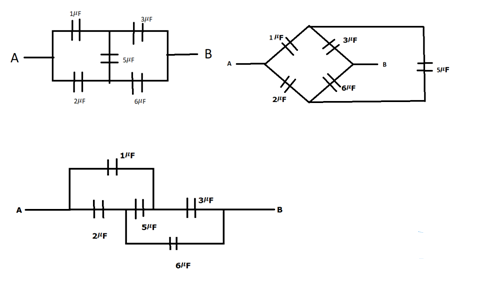# Convince yourself that parts (a), (b), (c)`
Question:

Convince yourself that parts (a), (b), (c) of figure are identical. Find the capacitance between the points A and B of the assembly.Solution:

All the circuits are in balanced wheat stone symmetry so no current flows is $5 \mu \mathrm{F}$ capacitor

$C_{e q}=\frac{1 \times 3}{1+3}+\frac{2 \times 6}{2+6}=2.25 \mu F$